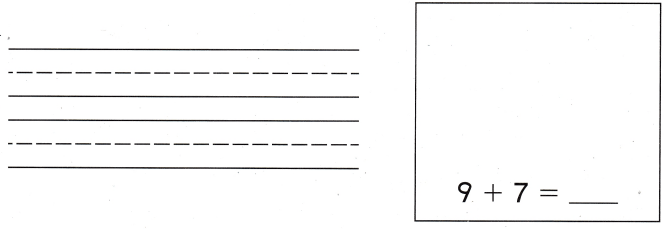Refer to our Texas Go Math Grade 1 Answer Key Pdf to score good marks in the exams. Test yourself by practicing the problems from Texas Go Math Grade 1 Lesson 8.2 Answer Key Generate Addition Word Problems.

Essential Question
Explanation:
8 + 2 = 10
Rosy catches 8 birds and then he catches 2 more birds.

Explore
4 + 3 = ____
Draw to show a story problem. Then solve.

4 + 3 = ___
Explanation:
4 + 3 = 7
Diana has 4 balloons and her sister gave 3 more balloons. How many balloons does Diana have now.For The Teacher

• Have children tell a story problem using the addition fact. Then have them use two-color counters to solve the problem. Have them draw the counters to show their work.The story problem about the picture.
. Kira has 8 computer games. There are 8 adventure games. how many games are there all together.
8 + 8 = 16

Math Talk
Mathematical processes
Tell your story problem to a classmate. How are your story problems alike?
Explanation:
Mary sees 8 squirrels. Jack sees 9 more squirrels than Mary.
How many squirrels does Jack see?
8 + 9 = 17 squirrels that jack see
This is my friends story problem.

Model and Draw

Write a story problem for the fact 5 + 3. Draw a picture to solve.Explanation: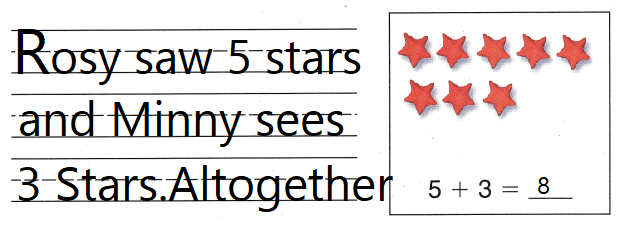Share and Show

Write a story problem for the addition fact. Draw a picture to solve.

Question 1.
9 + 4 = ___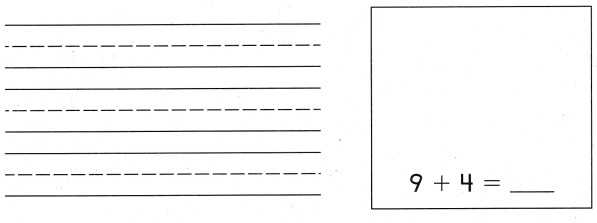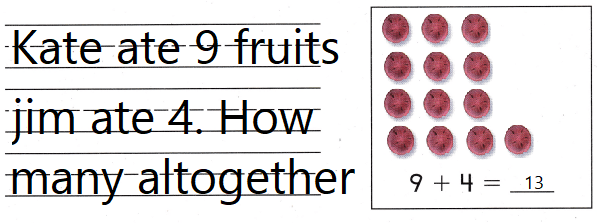Explanation:
Written a story problem for addition fact.

Problem Solving

Write a story problem for the addition fact. Draw a picture to solve.

Question 2.
H.O.T. 5 + ___ = 9Question 3.
H.O.T. Multi-Step ____ + ____ = 15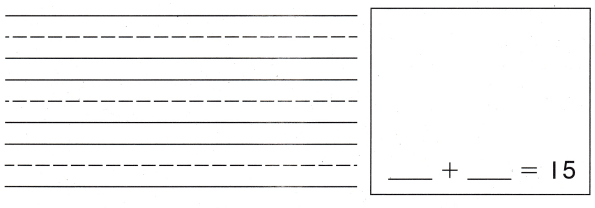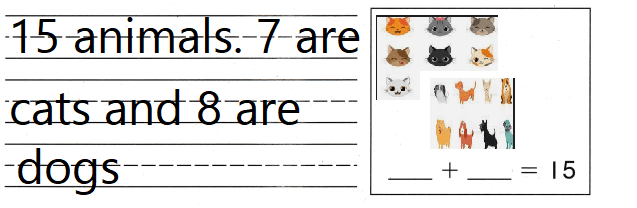Explanation:
Written  a story problem for 7 +8 = 15

Question 4.
Which addition fact matches the drawing?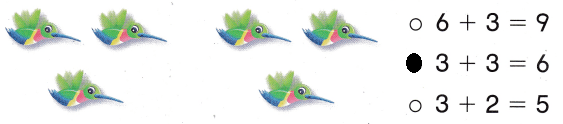Explanation:
3 + 3 = 6
is the addition fact of the picturs

Question 5.
Which drawing matches the addition fact?
4 + 3 = __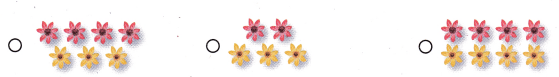Question 6.
Use Tools Cody made this story problem for 6 + 5 = ___. 6 cows are on a hill. How many cows are on the hill now? Which sentence did Cody forget to write in the story problem?
(A) 5 cows join them.
(B) 5 cows walk away.
(C) 5 horses go up the hill.
Explanation:
6 + 5 = 11
6 cows are on a hill. 5 cows join them.

Question 7.
Texas Test Prep Which addition sentence matches the model?(A) 5 + 3 = 8
(B) 8 + 8 = 16
(C) 6 + 8 = 14
Explanation:
6 + 8 = 14
Matches the sentence.

Take Home Activity

• Have your child write a story problem for 7 + 4. Then have him or her draw o picture to solve the problem.
Explanation:
Zima has 6 toy cars. Gaby has 9 more toy cars than Zima.6 + 9 = 15.

### Texas Go Math Grade 1 Lesson 8.2 Homework and Practice Answer Key

Write a story problem for the addition fact.

Draw a picture to solve.

Question 1.
6 + __ = 11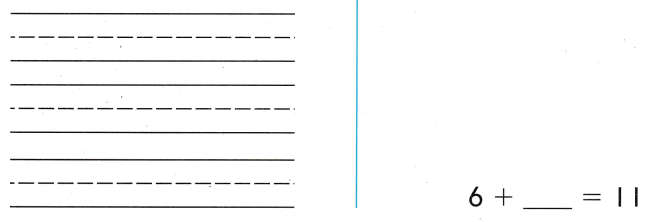Explanation:
Written a story problem for 6 + 5 = 11

Problem Solving

Write a story problem for the addition fact. Draw a picture to solve.

Question 2.
____ + ___ = 17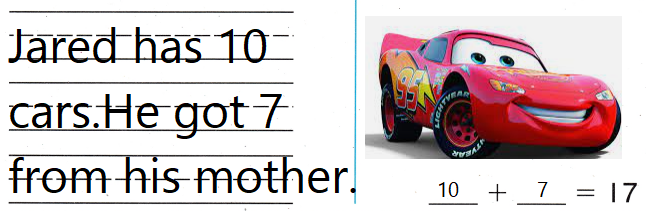Explanation:
Written a story problem for 10 + 7 = 17

Texas Test Prep

Lesson Check

Question 3.
Which addition fact matches the drawing?(A) 5 + 4 = 9
(B) 2 + 2 = 4
(C) 8 + 1 = 9
Explanation:
5 + 4 = 9 matches the addition fact.

Question 4.
Which drawing matches the addition fact?
4 + 8 = ___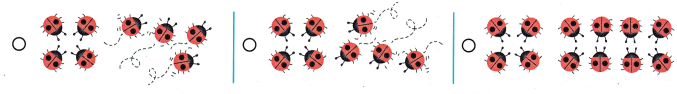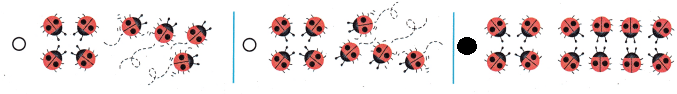Explanation:
4 + 8 = 12
Bubbled the drawing which matches the addition fact.

Question 5.
Which addition sentence matches the counters?(A) 7 + 7 = 14
(B) 6 + 8 = 14
(C) 5 + 9 = 14
Explanation:
5 + 9 = 14